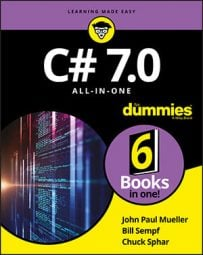##### C# 7.0 All-in-One For DummiesBoth the integer and floating-point types have their problems in C#. Floating-point variables have rounding problems associated with limits to their accuracy, while `int` variables just lop off the fractional part of a variable. In some cases, you need a variable type that offers the best of two worlds:
• Like a floating-point variable, it can store fractions.
• Like an integer, numbers of this type offer exact values for use in computations — for example, 12.5 is really 12.5 and not 12.500001.
Fortunately, C# provides such a variable type, called `decimal`. A `decimal` variable can represent a number between 10–28 and 1028 — which represents a lot of zeros! And it does so without rounding problems.

## Declaring a decimal

Decimal variables are declared and used like any variable type, like this:

`decimal m1 = 100; // Good`

`decimal m2 = 100M; // Better`

The first declaration shown here creates a variable `m1` and initializes it to a value of `100`. What isn’t obvious is that 100 is actually of type `int`. Thus, C# must convert the `int` into a `decimal` type before performing the initialization. Fortunately, C# understands what you mean — and performs the conversion for you.

The declaration of `m2` is the best. This clever declaration initializes `m2` with the `decimal` constant 100M. The letter M at the end of the number specifies that the constant is of type `decimal`. No conversion is required.

## Comparing decimals, integers, and floating-point types

The `decimal` variable type seems to have all the advantages and none of the disadvantages of `int` or `double` types. Variables of this type have a very large range, they don’t suffer from rounding problems, and 25.0 is 25.0 and not 25.00001.

The `decimal` variable type has two significant limitations, however. First, a `decimal` is not considered a counting number because it may contain a fractional value. Consequently, you can’t use them in flow-control loops.

The second problem with `decimal` variables is equally serious or even more so. Computations involving `decimal` values are significantly slower than those involving either simple integer or floating-point values. On a crude benchmark test of 300,000,000 adds and subtracts, the operations involving `decimal` variables were approximately 50 times slower than those involving simple `int` variables. The relative computational speed gets even worse for more complex operations. Besides that, most computational functions, such as calculating sines or exponents, are not available for the `decimal` number type.

Clearly, the `decimal` variable type is most appropriate for applications such as banking, in which accuracy is extremely important but the number of calculations is relatively small.